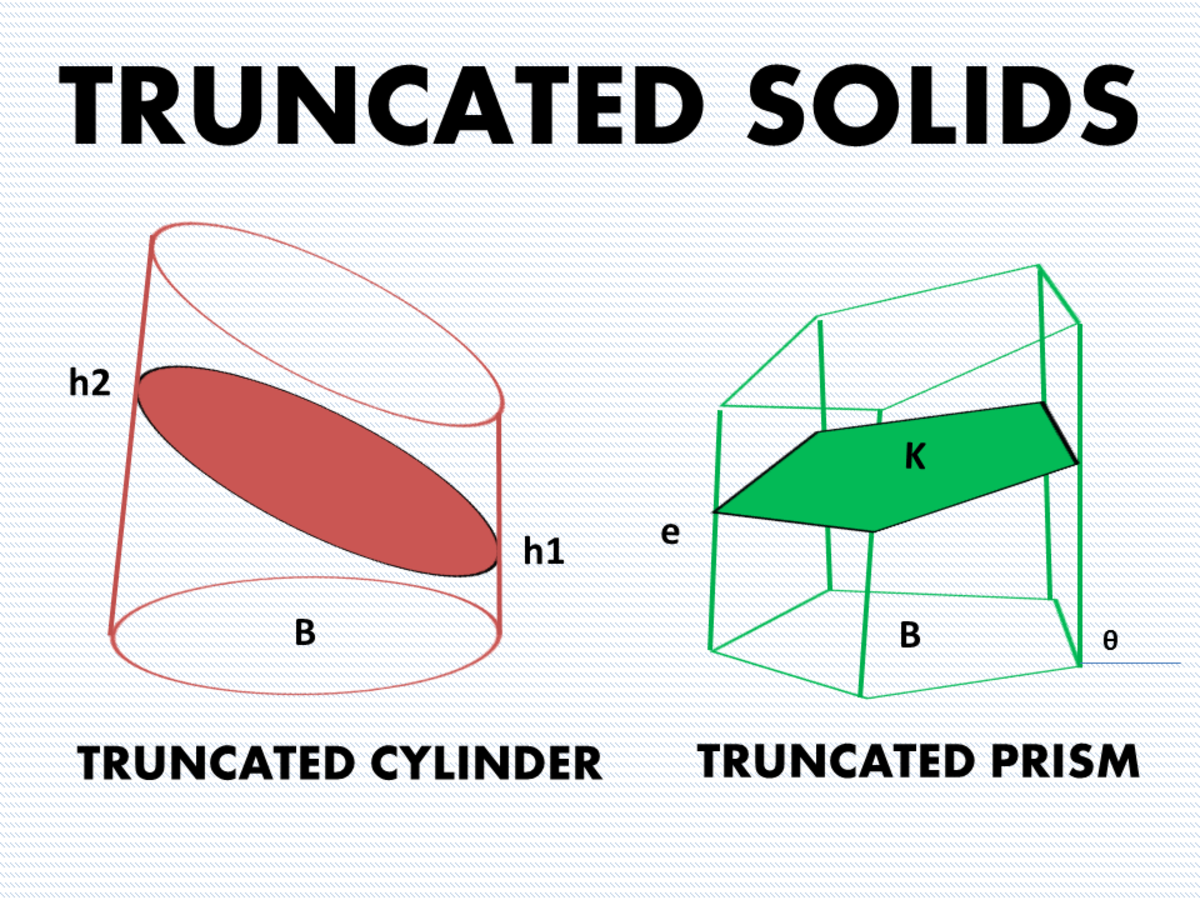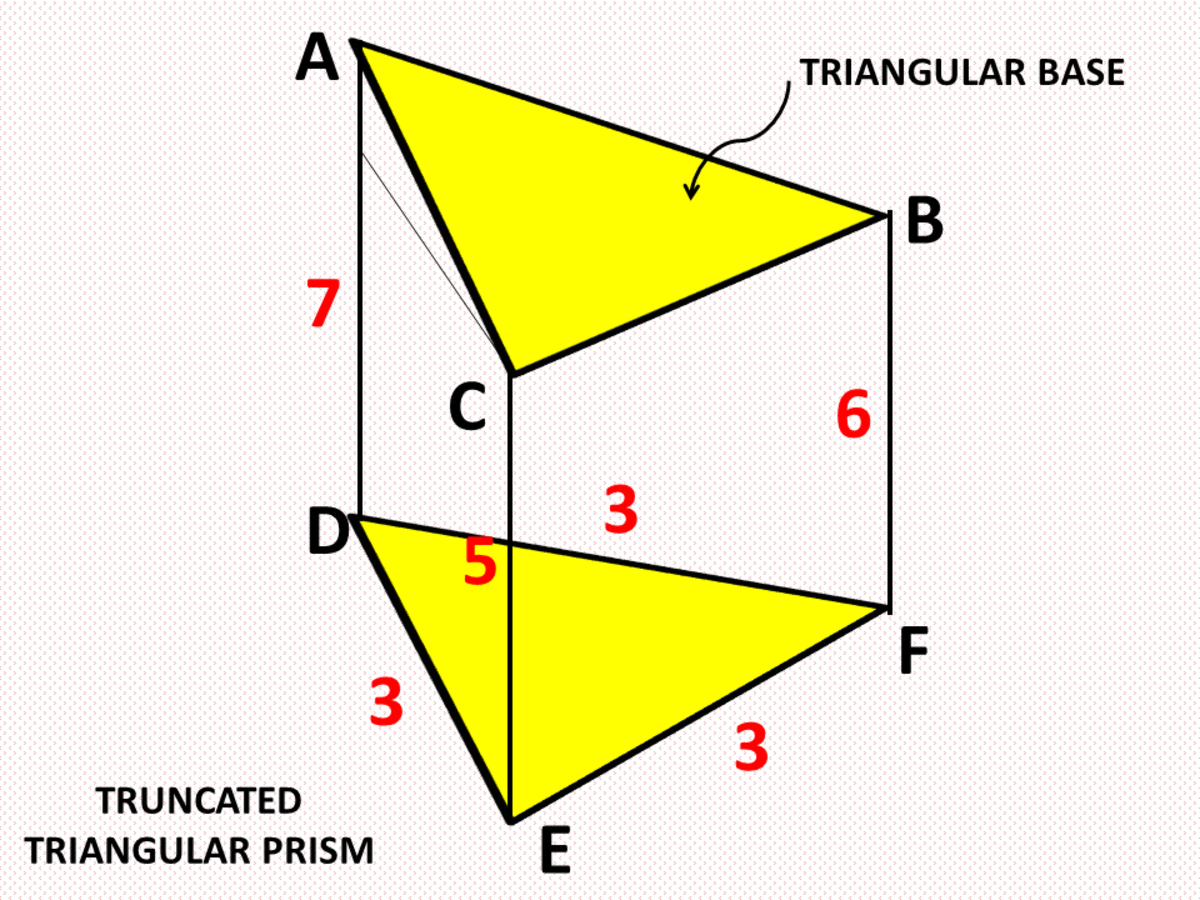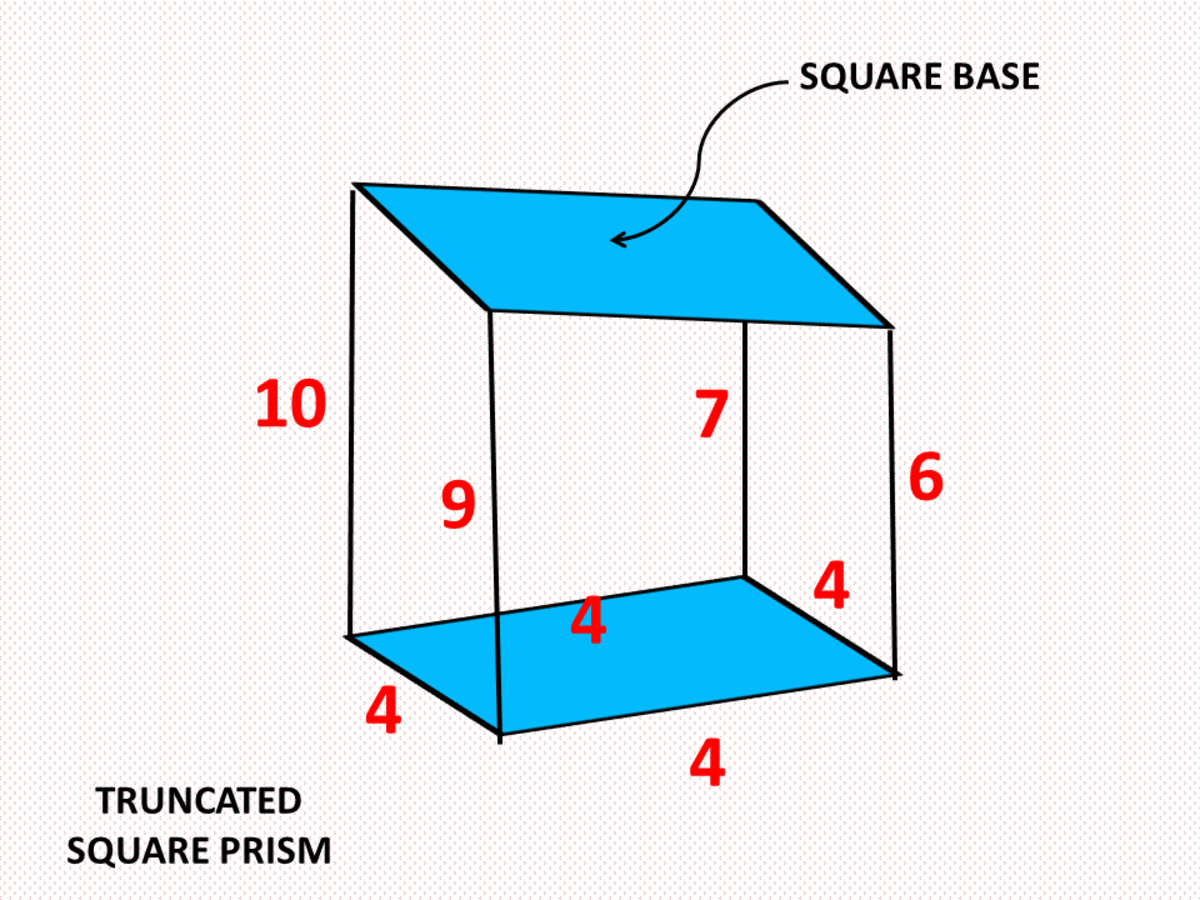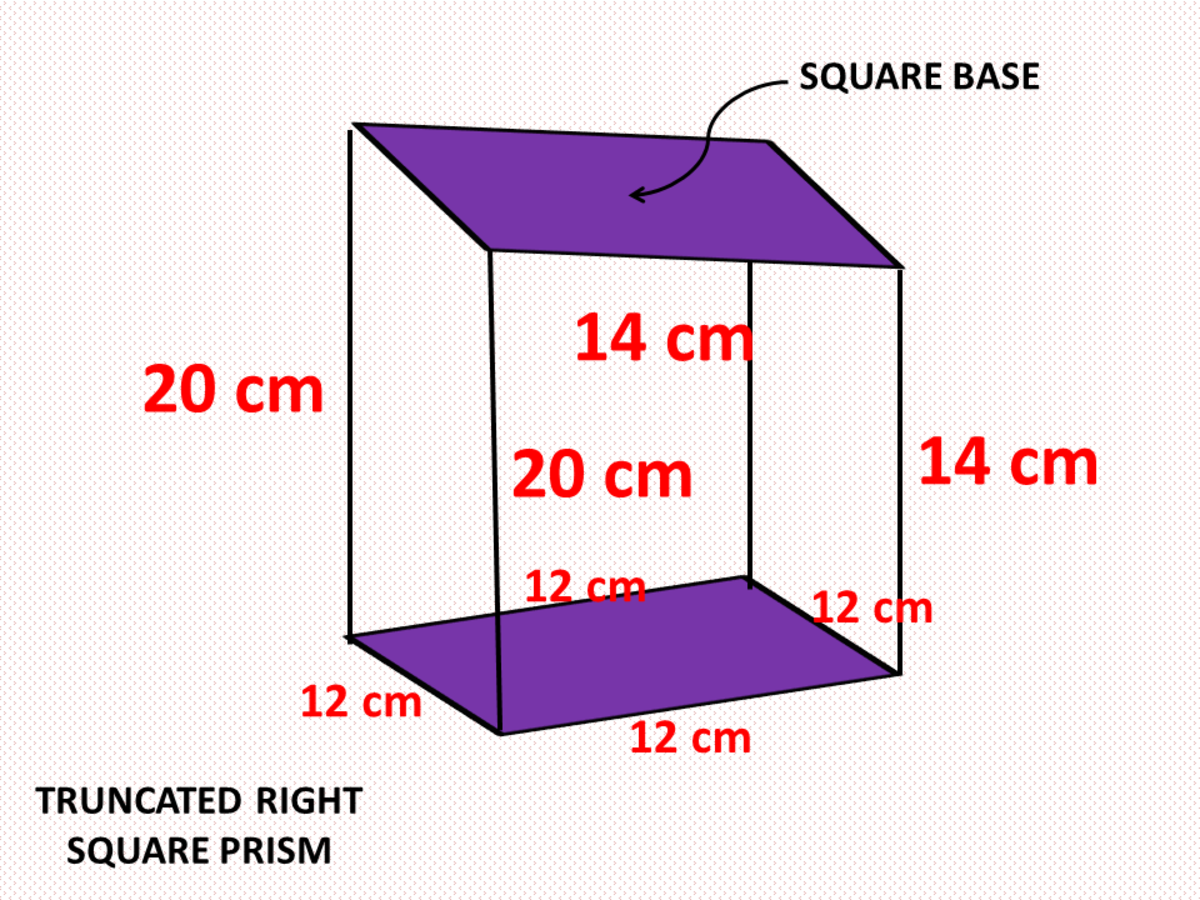# Finding the Surface Area and Volume of Truncated Cylinders and Prisms

• Author:
• Updated date:

Ray is a licensed engineer in the Philippines. He loves to write about mathematics and civil engineering.Finding the Surface Area and Volume of Truncated Cylinders and PrismsJohn Ray Cuevas

## What Is a Truncated Cylinder?

A truncated circular cylinder, also known as the cylindrical segment, is a solid formed by passing a non parallel plane through a circular cylinder. The noncircular upper base is tilted to the circular section. If the circular cylinder is a right cylinder, then every right section is a circle having the same area as the base.

Let K be the area of the right section and h1 and h2 the shortest and longest element of the truncated cylinder, respectively. The volume of the truncated circular cylinder is given by the formula below. If the truncated cylinder is a right circular cylinder of radius r, the volume can be expressed in terms of the radius.

V = K [(h1 + h2)/2]

V = πr2 [(h1 + h2)/2]

## What Is a Truncated Prism?

A truncated prism is a portion of a prism formed by passing a plane not parallel to the base and intersecting all the lateral edges. Since the truncating plane is not parallel to the base, the solid formed has two nonparallel bases, which are both polygons of the same number of edges. The lateral edges are non-congruent and the lateral faces are quadrilaterals (rectangles or trapezoids). If the cut off prism is a right prism, then the lateral faces are right trapezoids. The total surface area of a truncated prism is the sum of the areas of the two polygonal bases and the right trapezoidal faces.

In general, the volume of a truncated prism is equal to the product of the area of its right section, and the average of the lengths of its lateral edges. K is the area of the right section and L is the average length of the lateral edges. For a truncated regular prism, the right section is equal to the base area. The volume of a truncated prism is given by the formula below. K is B multiplied by the value of sinθ, L is equal to the average length of its lateral edges, and n is the number of sides of the base.

V = KL

V = BL

## Problem 1: Surface Area and Volume of a Truncated Triangular Prism

A truncated right prism has an equilateral triangular base with one side that measures 3 centimeters. The lateral edges have lengths of 5 cm, 6 cm, and 7 cm. Find the total surface area and the volume of the truncated right prism.Surface Area and Volume of a Truncated Triangular PrismJohn Ray Cuevas

### Solution

a. Since it is a right truncated prism, all lateral edges are perpendicular to the lower base. This make each lateral face of the prism a right trapezoid. Compute for the edges AC, AB, and BC of the upper base using the given measures in the problem.

AC = √32 + (7 - 5)2

Scroll to Continue

AC = √13 centimeters

AB = √32 + (7 - 6)2

AB = √10 centimeters

BC = √32 + (6 - 5)2

AB = √10 centimeters

b. Compute for the area of triangle ABC and triangle DEF using Heron's formula.

s = (a + b + c)/2

s = (√13 + √10 + √10)/2

s = 4.965

AABC = √4.965(4.965 - √13)(4.965 - √10)(4.965 - √10)

AABC = 4.68 cm2

c. Compute for the area of the trapezoidal faces.

AACED = 1/2 (7 +5) (3)

AACED = 18 cm2

ABCEF = 1/2 (6 + 5) (3)

ABCEF = 16.5 cm2

AABFD = 1/2 (7 +6) (3)

AABFD = 19.5 cm2

d. Solve for the total surface area of the truncated prism by summing up all areas.

TSA = B1 + B2 + LSA

TSA = 4.68 + 3.90 + 18 +16.5 +19.5

TSA = 62.6 cm2

e. Solve for the volume of the truncated right prism.

V = BL

V = 3.90 [(7 + 6 +5)/3]

V = 23.4 cm3

Final Answer: The total surface area and volume of the truncated right prism given above are 62.6 cm2 and 23.4 cm3, respectively.

## Problem 2: Volume and Lateral Area of a Truncated Right Square Prism

Find the volume and the lateral area of a truncated right square prism whose base edge is 4 feet. The lateral edges measure 6 feet, 7 feet, 9 feet, and 10 feet.Volume and Lateral Area of a Truncated Right Square PrismJohn Ray Cuevas

### Solution

a. Since it is a right truncated square prism, all lateral edges are perpendicular to the lower base. This make each lateral face of the prism a right trapezoid. Compute for the edges of the upper square base using the given measures in the problem.

S1 = √42 + (10 - 9)2

S1 = √17 feet

S2 = √42 + (9 - 6)2

S2 = 5 feet

S3 = √42 + (7 - 6)2

S3 = √17 feet

S4 = √42 + (10 - 7)2

S4 = 5 feet

b. Compute for the area of the trapezoidal faces.

A1 = 1/2 (10 + 9) (4)

A1 = 38 ft2

A2= 1/2 (9 + 6) (4)

A2= 30 ft2

A3 = 1/2 (7 +6) (4)

A3 = 26 ft2

A4 = 1/2 (7 + 10) (4)

A4 = 34 ft2

c. Compute the total lateral area by getting the sum of all the areas of the lateral faces.

TLA = A1 + A2 + A3 + A4

TLA = 38 + 30 + 26 + 34

TLA = 128 ft2

e. Solve for the volume of the truncated right square prism.

V = BL

V = 42 [(6 + 7 + 9 + 10)/4]

V = 128 ft3

Final Answer: The total surface area and volume of the truncated right square prism given above are 128 ft2 and 128 ft3, respectively.

## Problem 3: Volume of a Right Circular Cylinder

Show that the volume of a truncated right circular cylinder is V = πr2 [(h1 + h2) / 2].

### Solution

a. Simplify all variables of the given formula for volume. B denotes the area of the base, and h1 and h2 denote the shortest and longest elements of the truncated cylinder shown above.

B = area of the circular base

B = πr2

b. Partition the truncated cylinder into two solids such that the wedge part has a volume equal to one-half the volume of the upper cylinder with height h2 - h1. The volume of the upper cylinder is denoted by V1. On the other hand, the lower part is a cylinder with altitude h1 and volume V2.

V = (1/2) V1 + V2

V1 = B(h2 - h1)

V2 = B x h1

V = (1/2)(B(h2 - h1)) + (B x h1)

V = (1/2)(B x h2) - (1/2)(B x h1) + (B x h1)

V = B [(h1 + h2)/2]

V = πr2 [(h1 + h2)/2]

Final Answer: The volume of a truncated right circular cylinder is V = πr2 [(h1 + h2)/2].

## Problem 4: Total Surface Area of a Truncated Right Square Prism

A block of the earth in the form of a truncated right prism has a square base with edges measured 12 centimeters. Two adjacent lateral edges are each 20 cm long, and the other two lateral edges are each 14 cm long. Find the total surface area of the block.Total Surface Area of a Truncated Right Square PrismJohn Ray Cuevas

### Solution

a. Since it is a right truncated square prism, all lateral edges are perpendicular to the lower base. This make each lateral face of the prism a right trapezoid. Compute for the edges of the upper square base using the given measures in the problem.

S1 = √122 + (20 - 20)2

S1 = 12 centimeters

S2 = √122 + (20 - 14)2

S2 = 6√5 centimeters

S3 = √122 + (14 - 14)2

S3 = 12 centimeters

S4 = √122 + (20 - 14)2

S4 = 6√5 centimeters

b. Compute for the area of lower square base and the upper rectangular base.

AUPPER = 12 x 6√5

AUPPER = 72√5 cm2

ALOWER = 12 x 12

ALOWER = 144 cm2

b. Compute for the area of the rectangular and trapezoidal faces of the truncated right square prism given.

A1 = 20 x 12

A1 = 240 cm2

A2= 1/2 (20 + 14) (12)

A2= 204 cm2

A3 = 14 x 12

A3 = 168 cm2

A4 = 1/2 (20 + 14) (12)

A4 = 204 cm2

d. Solve for the total surface area of the truncated square prism by summing up all areas.

TSA = AUPPER + ALOWER + LSA

TSA = 72√5 + 144 + 240 + 204 + 168 + 204

TSA = 1120.10 cm2

Final Answer: The total surface area of the given truncated square prism is 1120.10 cm2.

## Other Topics About Surface Area and Volume

Ray (author) from Philippines on January 27, 2020:

Thank you, Ms Chitrangada Sharan. I really love teaching math subjects!

Ray (author) from Philippines on January 27, 2020:

Thank you and welcome, Mr. Eric Dierker!

Chitrangada Sharan from New Delhi, India on January 27, 2020:

A well explained article and very helpful for students. I hope that they get benefitted by this.

You reminded me of my school days. Such articles are important and should be published online.

Thanks for sharing.

Eric Dierker from Spring Valley, CA. U.S.A. on January 26, 2020:

A bit above my understanding so I learned so new things, thank you.

Ray (author) from Philippines on January 26, 2020:

Thank you very much Mr. Umesh Chandra Bhatt.

It is my ultimate goal to provide students the easiest methods or ways to solve different math problems. I, myself had been in a situation where I almost hated and cursed my math subjects. I struggled in Math a lot during my primary and secondary school. Now, as a licensed Civil Engineer, I want students to realize that Math is actually one of the easiest and enjoying subjects to learn with proper guide and teaching.

Umesh Chandra Bhatt from Kharghar, Navi Mumbai, India on January 26, 2020:

Very useful for engineering snd science students. Well explained.